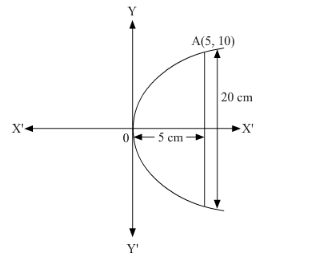# If a parabolic reflector is 20 cm in diameter and 5 cm deep, find the focus.`
Question:

If a parabolic reflector is 20 cm in diameter and 5 cm deep, find the focus.

Solution:

The origin of the coordinate plane is taken at the vertex of the parabolic reflector in such a way that the axis of the reflector is along the positive x-axis.

This can be diagrammatically represented asThe equation of the parabola is of the form $y^{2}=4 a x$ (as it is opening to the right). Since the parabola passes through point $A$ ( 5,10 ),

$10^{2}=4 a(5)$

$\Rightarrow 100=20 a$

$\Rightarrow a=\frac{100}{20}=5$

Therefore, the focus of the parabola is (a, 0) = (5, 0), which is the mid-point of the diameter.

Hence, the focus of the reflector is at the mid-point of the diameter.